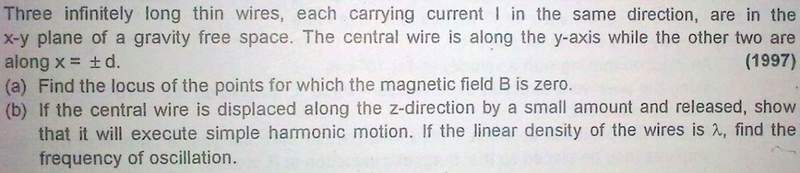# Mag field due to long wire

cupid.callin

## Homework StatementI have solved the question ... answer comes +-d/√3

but i think that field will also be 0 along Y-axis ...
but its not given in answer ...

Some help please ...

## Answers and Replies

zorro
As you approach the middle wire, the magnetic field due to it increases unbounded and that due to other two wires tends to 0. In short, the magnetic field at the middle wire is undefined.

Note that the question mentions 'thin wire' (radius tends to 0)

cupid.callin
are you sure?

because according to ampere circuital law ... when you draw a closed loop around the center of wire, just at the center ... inet will be 0 ... so B is 0

zorro
As I said, the wire is thin (radius tends to 0). So you don't need to worry about its thickness. If it was thick enough, then you are correct but the question clearly mentions that it is not so.

cupid.callin
it doesnt mater .. .if radius tends to 0 then the radius of loop also tends to 0

zorro
But Ampere's Law is not valid for loops of changing radius.
To add, the loop does not remain a loop when its radius tends to 0. It becomes a point.

cupid.callin
but this explanation is not satisfactory

zorro
Lets put it another way.
The cross-section of the wire is not defined (which is not possible in reality offcourse, such assumptions for 'thin wires' are valid in problems). So you cannot draw an Amperian loop inside because there is no such 'inside'.

Phrak
The magnetic field strength B goes to infinity for a wire with zero diameter,
but this is not the case for a small diameter wire. Implicitly, small diameter
wire means small with respect to the distance between wires.

The object of this lesson is to find the fields surrounding the wires. You should
be able to convince yourself that for the two wires at x = +d, and x= -d that
the field contributions from both wires is zero at x=0. But the problem you are
given is to find all the points in the x-y plane where B=0.

cupid.callin
But the problem you are given is to find all the points in the x-y plane where B=0.

No, i am asked to find the locus of point where B=0, in any plane

and also Y axis in in XY plane ... its x=0,z=0 ... which is a line in XY plane

@Abdul
i guess Phrak is right about the definition of thin in the question ... if we talk about wire with radius=0 ... how will there be any current through it? ... if there is place for current to pass then of course there will be a place to draw a gaussian surface

cupid.callin
help someone ...

Phrak
No, i am asked to find the locus of point where B=0, in any plane

You're correct. I meant the X-Z plane. The field values are independent of Z.

There are three points in the X-Z plane. Can you name them?

zorro
i guess Phrak is right about the definition of thin in the question ...

He might be correct. But I don't interpret the question in that way. The magnetic field is undefined at the centre of the middle wire.

As I said before, if you consider the wire to be thick enough (small diameter as Phrak says), then the net magnetic field should be 0 all along the Y-axis. This is not included in the answer so I think radius ----> 0 is a possible interpretation.

if we talk about wire with radius=0 ... how will there be any current through it? ... if there is place for current to pass then of course there will be a place to draw a gaussian surface

I never said radius=0, I said radius tends to 0cupid.callin
You're correct. I meant the X-Z plane. The field values are independent of Z.

There are three points in the X-Z plane. Can you name them?

i cant get you

cupid.callin
I never said radius=0, I said radius tends to 0Even if radius --> 0 ... there will be some place through which current will travel ... it cant travel inside a simple line .... reember i=q/t ... q is charge crossing any surface perpendicular to wire in time t

if radius ---> 0
area --> 0
so how will there be current?

zorro
You need just an electron for current to flow. Imagine the wire with very very very small radius upto the radius of an electron (which can be approximated to --->0). Things become unreal at this stage. I had a similar doubt and I asked this to my Professor some months back. He replied with all the points I gave above. He said that the magnetic field is undefined at the centre. Afraid thats all I have got for you.

Think about this - The question says infinite wires. Do you have an infinite source of charge? (imaginary!)

Phrak
i cant get you

Move your perspective the X-Z plane. The wires are represented as
dots at x=-d, x=0, and x=d.

B, for each wire, is a vector tangent to a circle around the wire
and inversely proportional to the distance from the wire. Where in the
plane do the fields cancel? That is, find the locus of points B(x,z)=0.